Orbital eccentricity

Orbital eccentricity is the measure of the departure of an orbit from a perfect circle.

Definitions

In geometry, eccentricity (e) is a concept universally applicable to conic sections.

For the general case of an ellipse having semi-major axis a and distance c from the center to either focus: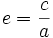A circle is a "degenerate" ellipse. In a circle, the two foci converge at the center. Thereforeand.

A parabola is an extreme case of an ellipse and is the first open conic section. For any parabola:Therefore, for any closed orbit,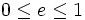Practical application

In astrodynamics, any given pair of apsides can predict the semi-major axis and eccentricity of any orbit. Specifically, for periapsis q and apoapsis Q: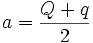or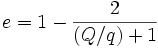By the same token, a and e can predict Q and q.and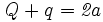Therefore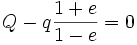andSubtracting the first equation from the second yields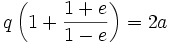From the above:andFor,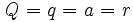, the orbital radius, as one would expect.